# How to Find Factorial of a Number Using RecursionRecursion is maybe the easiest yet most problematic concept for the beginners to grasp. But you will get hand of this concept after this Tech-Recipe. Click on “Continue Reading” to read about Recursion and a very easy example.

This tech-recipe will introduce you to the concept of recursion. With the simple example of Factorial of a Number Using Recursion.

### What is Recursion

Recur simply means to reoccur or to happen again. Recursion is a major programming concept in Computer science. Recursion is a process in which function calls itself. The function thus would be called a recursive function.

Sometimes it is easier to define the problem in terms of the problem itself. Especially for the problems that can be broken down into simpler versions

### Example:

Factorial Problems

### 6!=6x5x4x3x2x1! —–> 12

Now this problem can be broken down into simpler versions for itself.

We could write

### 6!=6×5! —–> 12

In general, we can express the factorial function as follows:

### n! = n * (n-1)!

Is this correct? Well… almost.

The factorial function is only defined for positive integers. So we should be a bit more precise:

### n! = 1 (if n is equal to 1) n! = n * (n-1)! (if n is larger than 1)

For certain problems, a recursive solution often leads to short and elegant code.
Compare the recursive solution with the iterative solution:

###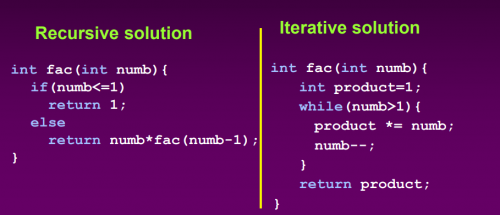Here’s how you can write a Recursive solution of Factorial Problem

Step-by-Step Procedure:

### Factorial of a Number Using Recursion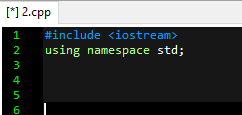2. Make a function that will receive an integer parameter and will return an integer. [So a parameter can be passed to this function. This function will compute the factorial and will return it.]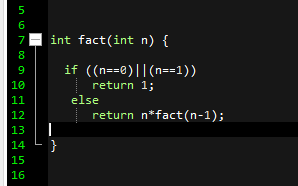3. Write the code that will calculate the factorial.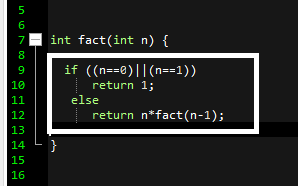4. A recursive function always has to bottom out. i.e. There will be a final result where the recursion can stop. Otherwise, recursion will continue forever. So make sure you write it’s bottom out.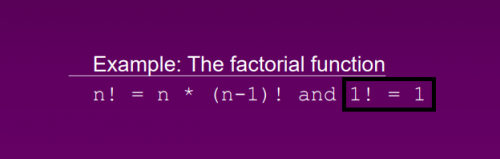### Note

A recursive function must contain at least one non-recursive branch.
The recursive calls must eventually lead to non-recursive branch.

5. Make a main function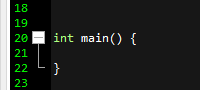6. Make initialize and declare an integer.

7. Call the factorial function in the main and pass the integer.

8. Be a fancy programmer and print the returned result neatly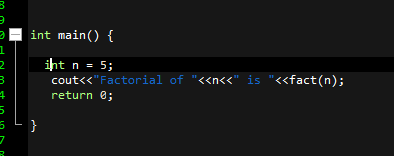And there you have it. You’re first recursive program. Practice this and other questions.

### Points to keep in mind

1. If we use iteration, we must be careful not to create an infinite loop by accident
2. If we use recursion we must be careful not to create an infinite chain of function calls
3. Always make sure that the recursion bottoms out